PHY_10_37_V1_P_Capacitors
Оценка 4.6

# PHY_10_37_V1_P_Capacitors

Оценка 4.6
pptx
07.05.2020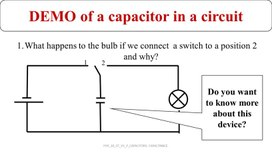PHY_10_37_V1_P_Capacitors.pptx

DEMO of a capacitor in a circuit

What happens to the bulb if we connect a switch to a position 2 and why?

PHY_10_37_V1_P_CAPACITORS. CAPACITANCE.

Capacitor and its Capacitance

10.3.1.4 to explain the role of capacitors in a simple electrical circuit

Learning objectives

PHY_10_37_V1_P_CAPACITORS. CAPACITANCE.

1.What is a capacitor? How does it made up? Types of capacitors
2. How a capacitor works in a circuit
3. Capacitance. Units of the capacitance.
4. Capacitance depends on geometry and insulation
5. Application of the capacitor

Group work activity

PHY_10_37_V1_P_CAPACITORS. CAPACITANCE.

## What is a capacitor A Capacitor is a two parallel plates, separated by air or some other insulator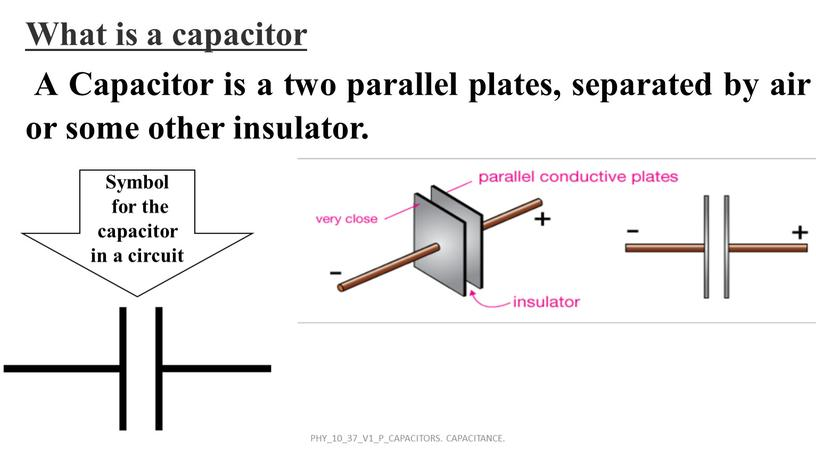What is a capacitor
A Capacitor is a two parallel plates, separated by air or some other insulator.

Symbol
for the capacitor in a circuit

PHY_10_37_V1_P_CAPACITORS. CAPACITANCE.

PHY_10_37_V1_P_CAPACITORS. CAPACITANCE.

## An example of a capacitor We get small distances by using very thin insulating materials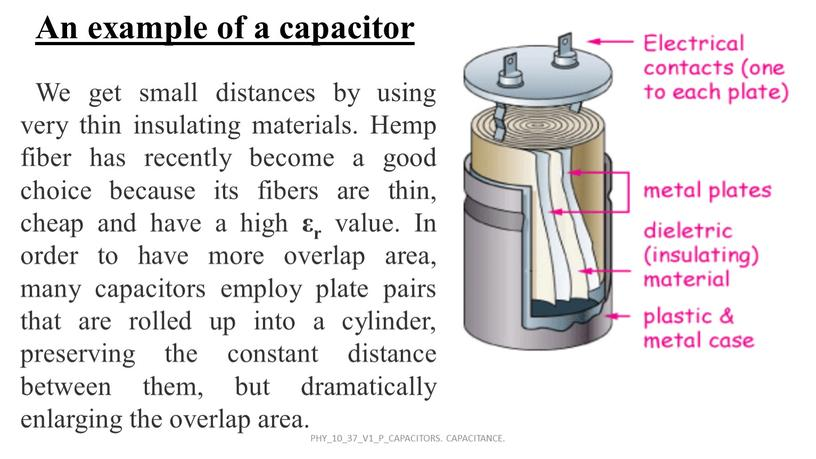An example of a capacitor

We get small distances by using very thin insulating materials. Hemp fiber has recently become a good choice because its fibers are thin, cheap and have a high εr value. In order to have more overlap area, many capacitors employ plate pairs that are rolled up into a cylinder, preserving the constant distance between them, but dramatically enlarging the overlap area.

PHY_10_37_V1_P_CAPACITORS. CAPACITANCE.

CAPACITORS

PHY_10_37_V1_P_CAPACITORS. CAPACITANCE.

PHY_10_37_V1_P_CAPACITORS. CAPACITANCE.

PHY_10_37_V1_P_CAPACITORS. CAPACITANCE.

DEMO of a capacitor in a circuit

Charge the capacitor;
Connect the voltmeter and measure the voltage;

PHY_10_37_V1_P_CAPACITORS. CAPACITANCE.

PHY_10_37_V1_P_CAPACITORS. CAPACITANCE.

Units of a capacitor

PHY_10_37_V1_P_CAPACITORS. CAPACITANCE.

## A capacitor that has air between its plates is connected across a potential difference of 12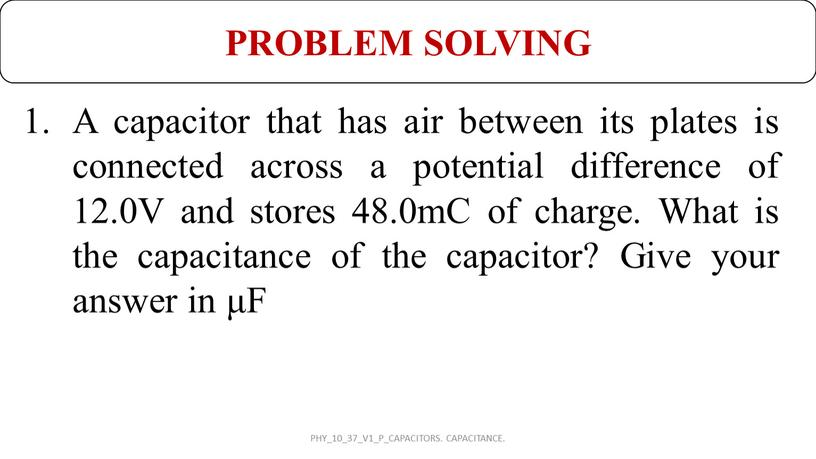A capacitor that has air between its plates is connected across a potential difference of 12.0V and stores 48.0mC of charge. What is the capacitance of the capacitor? Give your answer in μF

PROBLEM SOLVING

PHY_10_37_V1_P_CAPACITORS. CAPACITANCE.

## The amount of capacitance of such a device is dependent on three things :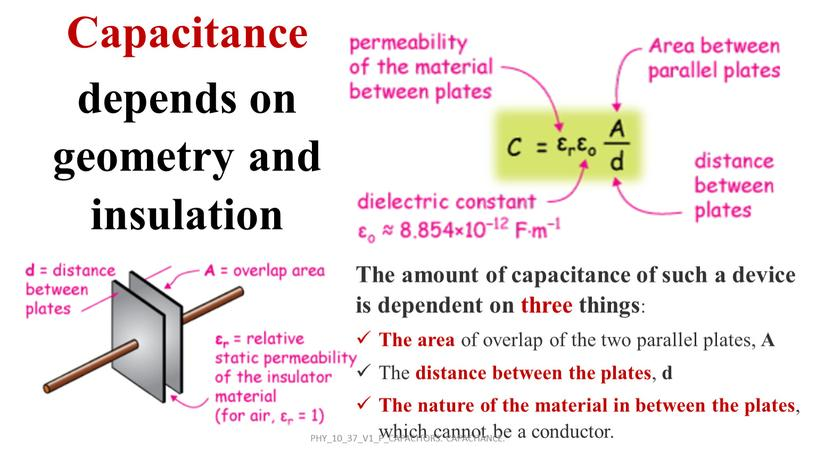The amount of capacitance of such a device is dependent on three things:
The area of overlap of the two parallel plates, A
The distance between the plates, d
The nature of the material in between the plates, which cannot be a conductor.

Capacitance
depends on geometry and insulation

PHY_10_37_V1_P_CAPACITORS. CAPACITANCE.

## We have a parallel plate capacitor constructed of two parallel plates, each with area 625 𝑐𝑚 2 625 𝑐𝑐𝑚𝑚 625 𝑐𝑚 2 2 625 𝑐𝑚…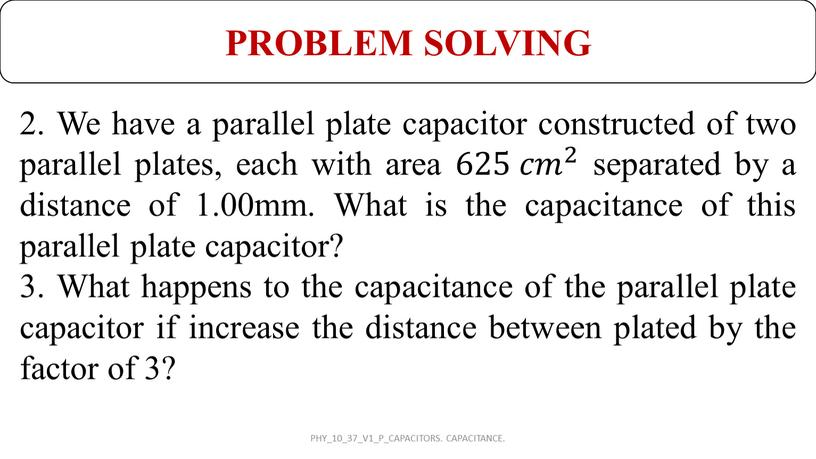2. We have a parallel plate capacitor constructed of two parallel plates, each with area 625 𝑐𝑚 2 625 𝑐𝑐𝑚𝑚 625 𝑐𝑚 2 2 625 𝑐𝑚 2 separated by a distance of 1.00mm. What is the capacitance of this parallel plate capacitor?
3. What happens to the capacitance of the parallel plate capacitor if increase the distance between plated by the factor of 3?

PROBLEM SOLVING

PHY_10_37_V1_P_CAPACITORS. CAPACITANCE.

PHY_10_37_V1_P_CAPACITORS. CAPACITANCE.

## Application of a capacitor PHY_10_37_V1_P_CAPACITORS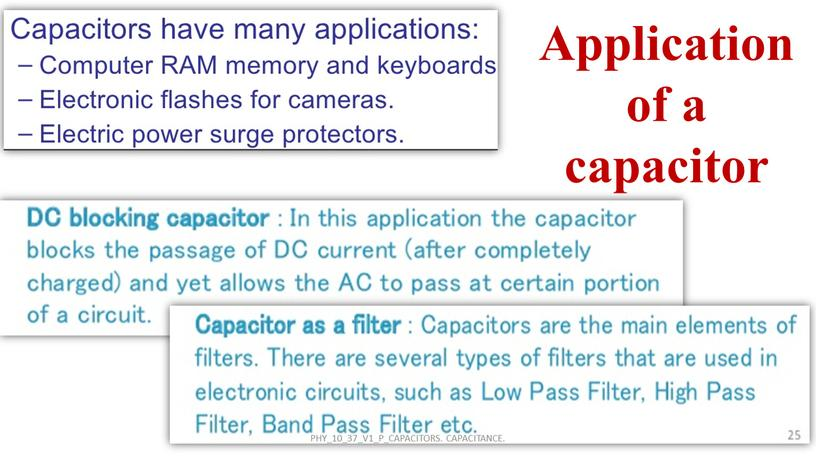Application of a capacitor

PHY_10_37_V1_P_CAPACITORS. CAPACITANCE.

## PROBLEM SOLVING 4. A parallel plate capacitor with air between the plates has a capacitance of 8pF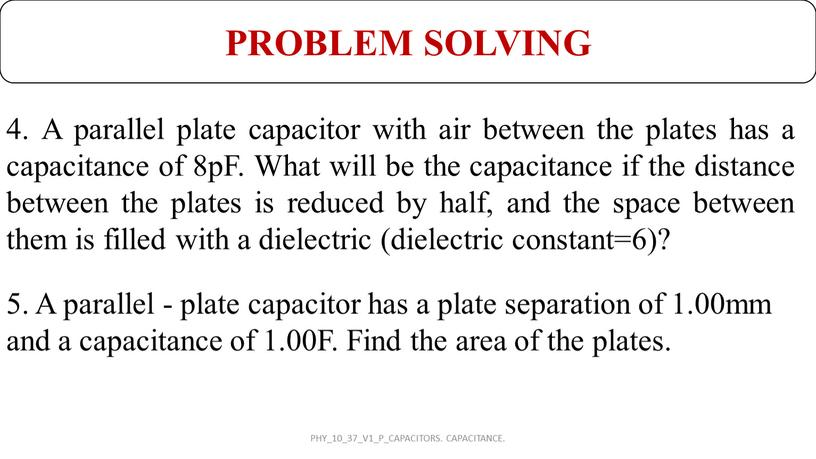PROBLEM SOLVING

4. A parallel plate capacitor with air between the plates has a capacitance of 8pF. What will be the capacitance if the distance between the plates is reduced by half, and the space between them is filled with a dielectric (dielectric constant=6)?

5. A parallel - plate capacitor has a plate separation of 1.00mm and a capacitance of 1.00F. Find the area of the plates.

PHY_10_37_V1_P_CAPACITORS. CAPACITANCE.

## Capacitor C1 consists of square parallel plates 10cm on side, and separated by a distance 4mm, as shown in the figure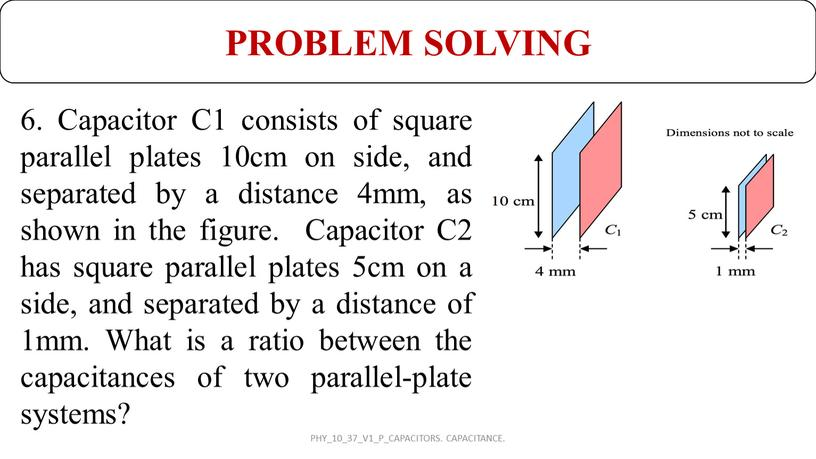6. Capacitor C1 consists of square parallel plates 10cm on side, and separated by a distance 4mm, as shown in the figure. Capacitor C2 has square parallel plates 5cm on a side, and separated by a distance of 1mm. What is a ratio between the capacitances of two parallel-plate systems?

PROBLEM SOLVING

PHY_10_37_V1_P_CAPACITORS. CAPACITANCE.

HOMEWORK

PHY_10_37_V1_P_CAPACITORS. CAPACITANCE.

Скачать файл